## 3.1 Defining the Derivative

### Learning Objectives

• Recognize the meaning of the tangent to a curve at a point.
• Calculate the slope of a tangent line.
• Identify the derivative as the limit of a difference quotient.
• Calculate the derivative of a given function at a point.
• Describe the velocity as a rate of change.
• Explain the difference between average velocity and instantaneous velocity.
• Estimate the derivative from a table of values.

Now that we have both a conceptual understanding of a limit and the practical ability to compute limits, we have established the foundation for our study of calculus, the branch of mathematics in which we compute derivatives and integrals. Most mathematicians and historians agree that calculus was developed independently by the Englishman Isaac Newton (1643–1727) and the German Gottfried Leibniz (1646–1716), whose images appear in (Figure). When we credit Newton and Leibniz with developing calculus, we are really referring to the fact that Newton and Leibniz were the first to understand the relationship between the derivative and the integral. Both mathematicians benefited from the work of predecessors, such as Barrow, Fermat, and Cavalieri. The initial relationship between the two mathematicians appears to have been amicable; however, in later years a bitter controversy erupted over whose work took precedence. Although it seems likely that Newton did, indeed, arrive at the ideas behind calculus first, we are indebted to Leibniz for the notation that we commonly use today.Figure 1. Newton and Leibniz are credited with developing calculus independently.

# Tangent Lines

We begin our study of calculus by revisiting the notion of secant lines and tangent lines. Recall that we used the slope of a secant line to a function at a point $(a,f(a))$ to estimate the rate of change, or the rate at which one variable changes in relation to another variable. We can obtain the slope of the secant by choosing a value of $x$ near $a$ and drawing a line through the points $(a,f(a))$ and $(x,f(x))$, as shown in (Figure). The slope of this line is given by an equation in the form of a difference quotient:

$m_{\sec}=\frac{f(x)-f(a)}{x-a}$.

We can also calculate the slope of a secant line to a function at a value $a$ by using this equation and replacing $x$ with $a+h$, where $h$ is a value close to $0$. We can then calculate the slope of the line through the points $(a,f(a))$ and $(a+h,f(a+h))$. In this case, we find the secant line has a slope given by the following difference quotient with increment $h$:

$m_{\sec}=\frac{f(a+h)-f(a)}{a+h-a}=\frac{f(a+h)-f(a)}{h}$.

### Definition

Let $f$ be a function defined on an interval $I$ containing $a$. If $x\ne a$ is in $I$, then

$Q=\frac{f(x)-f(a)}{x-a}$

is a difference quotient.

Also, if $h\ne 0$ is chosen so that $a+h$ is in $I$, then

$Q=\frac{f(a+h)-f(a)}{h}$

is a difference quotient with increment $h$.

View several Java applets on the development of the derivative.

These two expressions for calculating the slope of a secant line are illustrated in (Figure). We will see that each of these two methods for finding the slope of a secant line is of value. Depending on the setting, we can choose one or the other. The primary consideration in our choice usually depends on ease of calculation.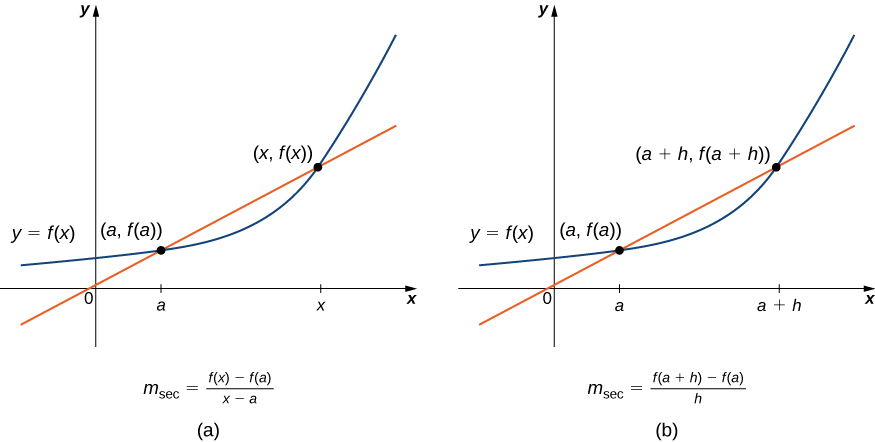Figure 2. We can calculate the slope of a secant line in either of two ways.

In (Figure)(a) we see that, as the values of $x$ approach $a$, the slopes of the secant lines provide better estimates of the rate of change of the function at $a$. Furthermore, the secant lines themselves approach the tangent line to the function at $a$, which represents the limit of the secant lines. Similarly, (Figure)(b) shows that as the values of $h$ get closer to 0, the secant lines also approach the tangent line. The slope of the tangent line at $a$ is the rate of change of the function at $a$, as shown in (Figure)(c).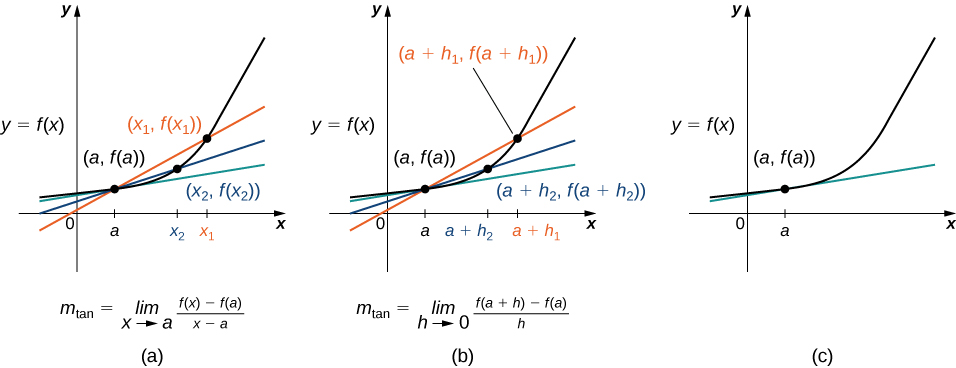Figure 3. The secant lines approach the tangent line (shown in green) as the second point approaches the first.

You can use this site to explore graphs to see if they have a tangent line at a point.

In (Figure) we show the graph of $f(x)=\sqrt{x}$ and its tangent line at $(1,1)$ in a series of tighter intervals about $x=1$. As the intervals become narrower, the graph of the function and its tangent line appear to coincide, making the values on the tangent line a good approximation to the values of the function for choices of $x$ close to 1. In fact, the graph of $f(x)$ itself appears to be locally linear in the immediate vicinity of $x=1$.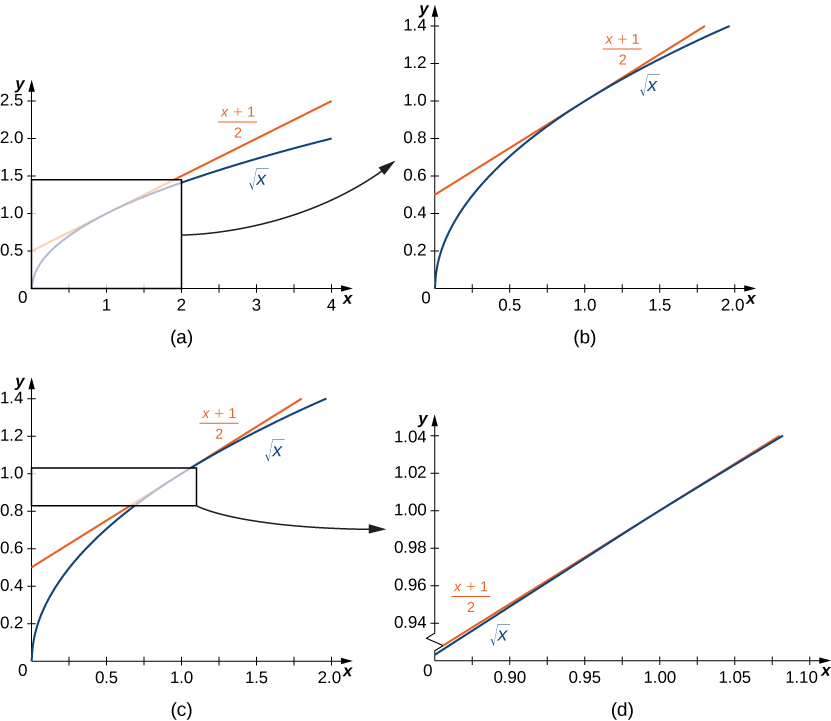Figure 4. For values of $x$ close to 1, the graph of $f(x)=\sqrt{x}$ and its tangent line appear to coincide.

Formally we may define the tangent line to the graph of a function as follows.

### Definition

Let $f(x)$ be a function defined in an open interval containing $a$. The tangent line to $f(x)$ at $a$ is the line passing through the point $(a,f(a))$ having slope

$m_{\tan}=\underset{x\to a}{\lim}\frac{f(x)-f(a)}{x-a}$

provided this limit exists.

Equivalently, we may define the tangent line to $f(x)$ at $a$ to be the line passing through the point $(a,f(a))$ having slope

$m_{\tan}=\underset{h\to 0}{\lim}\frac{f(a+h)-f(a)}{h}$

provided this limit exists.

Just as we have used two different expressions to define the slope of a secant line, we use two different forms to define the slope of the tangent line. In this text we use both forms of the definition. As before, the choice of definition will depend on the setting. Now that we have formally defined a tangent line to a function at a point, we can use this definition to find equations of tangent lines.

### Finding a Tangent Line

Find the equation of the line tangent to the graph of $f(x)=x^2$ at $x=3$.

### The Slope of a Tangent Line Revisited

Use (Figure) to find the slope of the line tangent to the graph of $f(x)=x^2$ at $x=3$.

### Finding the Equation of a Tangent Line

Find the equation of the line tangent to the graph of $f(x)=1/x$ at $x=2$.

Find the slope of the line tangent to the graph of $f(x)=\sqrt{x}$ at $x=4$.

#### Hint

Use either (Figure) or (Figure). Multiply the numerator and the denominator by a conjugate.

# The Derivative of a Function at a Point

The type of limit we compute in order to find the slope of the line tangent to a function at a point occurs in many applications across many disciplines. These applications include velocity and acceleration in physics, marginal profit functions in business, and growth rates in biology. This limit occurs so frequently that we give this value a special name: the derivative. The process of finding a derivative is called differentiation.

### Definition

Let $f(x)$ be a function defined in an open interval containing $a$. The derivative of the function $f(x)$ at $a$, denoted by $f^{\prime}(a)$, is defined by

$f^{\prime}(a)=\underset{x\to a}{\lim}\frac{f(x)-f(a)}{x-a}$

provided this limit exists.

Alternatively, we may also define the derivative of $f(x)$ at $a$ as

$f^{\prime}(a)=\underset{h\to 0}{\lim}\frac{f(a+h)-f(a)}{h}$
provided this limit exists.

### Estimating a Derivative

For $f(x)=x^2$, use a table to estimate $f^{\prime}(3)$ using (Figure).

For $f(x)=x^2$, use a table to estimate $f^{\prime}(3)$ using (Figure).

#### Hint

Evaluate $\frac{(x+h)^2-x^2}{h}$ at $h=-0.1,-0.01,-0.001,0.001,0.01,0.1$

### Finding a Derivative

For $f(x)=3x^2-4x+1$, find $f^{\prime}(2)$ by using (Figure).

### Revisiting the Derivative

For $f(x)=3x^2-4x+1$, find $f^{\prime}(2)$ by using (Figure).

For $f(x)=x^2+3x+2$, find $f^{\prime}(1)$.

#### Hint

Use either (Figure), (Figure), or try both. Use either (Figure) or (Figure) as a guide.

# Velocities and Rates of Change

Now that we can evaluate a derivative, we can use it in velocity applications. Recall that if $s(t)$ is the position of an object moving along a coordinate axis, the average velocity of the object over a time interval $[a,t]$ if $t>a$ or $[t,a]$ if $t<a$ is given by the difference quotient

$v_{\text{avg}}=\frac{s(t)-s(a)}{t-a}$.

As the values of $t$ approach $a$, the values of $v_{\text{avg}}$ approach the value we call the instantaneous velocity at $a$. That is, instantaneous velocity at $a$, denoted $v(a)$, is given by

$v(a)=s^{\prime}(a)=\underset{t\to a}{\lim}\frac{s(t)-s(a)}{t-a}$.

To better understand the relationship between average velocity and instantaneous velocity, see (Figure). In this figure, the slope of the tangent line (shown in red) is the instantaneous velocity of the object at time $t=a$ whose position at time $t$ is given by the function $s(t)$. The slope of the secant line (shown in green) is the average velocity of the object over the time interval $[a,t]$.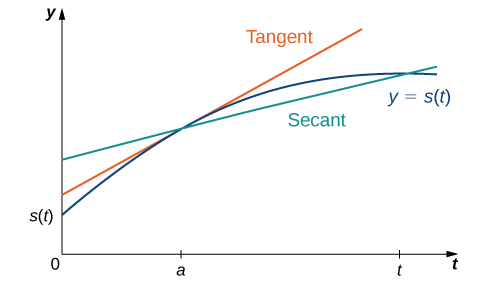Figure 7. The slope of the secant line is the average velocity over the interval $[a,t]$. The slope of the tangent line is the instantaneous velocity.

We can use (Figure) to calculate the instantaneous velocity, or we can estimate the velocity of a moving object by using a table of values. We can then confirm the estimate by using (Figure).

### Estimating Velocity

A lead weight on a spring is oscillating up and down. Its position at time $t$ with respect to a fixed horizontal line is given by $s(t)= \sin t$ ((Figure)). Use a table of values to estimate $v(0)$. Check the estimate by using (Figure).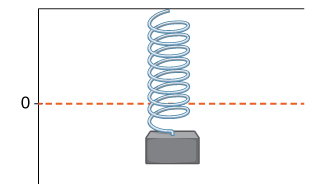Figure 8. A lead weight suspended from a spring in vertical oscillatory motion.

A rock is dropped from a height of 64 feet. Its height above ground at time $t$ seconds later is given by $s(t)=-16t^2+64, \, 0\le t\le 2$. Find its instantaneous velocity 1 second after it is dropped, using (Figure).

#### Hint

$v(t)=s^{\prime}(t)$. Follow the earlier examples of the derivative using (Figure).

As we have seen throughout this section, the slope of a tangent line to a function and instantaneous velocity are related concepts. Each is calculated by computing a derivative and each measures the instantaneous rate of change of a function, or the rate of change of a function at any point along the function.

### Definition

The instantaneous rate of change of a function $f(x)$ at a value $a$ is its derivative $f^{\prime}(a)$.

### Chapter Opener: Estimating Rate of Change of VelocityFigure 9. (credit: modification of work by Codex41, Flickr)

Reaching a top speed of 270.49 mph, the Hennessey Venom GT is one of the fastest cars in the world. In tests it went from 0 to 60 mph in 3.05 seconds, from 0 to 100 mph in 5.88 seconds, from 0 to 200 mph in 14.51 seconds, and from 0 to 229.9 mph in 19.96 seconds. Use this data to draw a conclusion about the rate of change of velocity (that is, its acceleration) as it approaches 229.9 mph. Does the rate at which the car is accelerating appear to be increasing, decreasing, or constant?

### Rate of Change of Temperature

A homeowner sets the thermostat so that the temperature in the house begins to drop from $70^{\circ}\text{F}$ at 9 p.m., reaches a low of $60^{\circ}$ during the night, and rises back to $70^{\circ}$ by 7 a.m. the next morning. Suppose that the temperature in the house is given by $T(t)=0.4t^2-4t+70$ for $0\le t\le 10$, where $t$ is the number of hours past 9 p.m. Find the instantaneous rate of change of the temperature at midnight.

### Rate of Change of Profit

A toy company can sell $x$ electronic gaming systems at a price of $p=-0.01x+400$ dollars per gaming system. The cost of manufacturing $x$ systems is given by $C(x)=100x+10,000$ dollars. Find the rate of change of profit when 10,000 games are produced. Should the toy company increase or decrease production?

A coffee shop determines that the daily profit on scones obtained by charging $s$ dollars per scone is $P(s)=-20s^2+150s-10$. The coffee shop currently charges $\3.25$ per scone. Find $P^{\prime}(3.25)$, the rate of change of profit when the price is $\3.25$ and decide whether or not the coffee shop should consider raising or lowering its prices on scones.

#### Hint

Use (Figure) for a guide.

### Key Concepts

• The slope of the tangent line to a curve measures the instantaneous rate of change of a curve. We can calculate it by finding the limit of the difference quotient or the difference quotient with increment $h$.
• The derivative of a function $f(x)$ at a value $a$ is found using either of the definitions for the slope of the tangent line.
• Velocity is the rate of change of position. As such, the velocity $v(t)$ at time $t$ is the derivative of the position $s(t)$ at time $t$. Average velocity is given by
$v_{\text{avg}}=\frac{s(t)-s(a)}{t-a}$.

Instantaneous velocity is given by

$v(a)=s^{\prime}(a)=\underset{t\to a}{\lim}\frac{s(t)-s(a)}{t-a}$.
• We may estimate a derivative by using a table of values.

# Key Equations

• Difference quotient
$Q=\frac{f(x)-f(a)}{x-a}$
• Difference quotient with increment $h$
$Q=\frac{f(a+h)-f(a)}{a+h-a}=\frac{f(a+h)-f(a)}{h}$
• Slope of tangent line
$m_{\tan}=\underset{x\to a}{\lim}\frac{f(x)-f(a)}{x-a}$
$m_{\tan}=\underset{h\to 0}{\lim}\frac{f(a+h)-f(a)}{h}$
• Derivative of $f(x)$ at $a$
$f^{\prime}(a)=\underset{x\to a}{\lim}\frac{f(x)-f(a)}{x-a}$
$f^{\prime}(a)=\underset{h\to 0}{\lim}\frac{f(a+h)-f(a)}{h}$
• Average velocity
$v_{\text{avg}}=\frac{s(t)-s(a)}{t-a}$
• Instantaneous velocity
$v(a)=s^{\prime}(a)=\underset{t\to a}{\lim}\frac{s(t)-s(a)}{t-a}$

For the following exercises, use (Figure) to find the slope of the secant line between the values $x_1$ and $x_2$ for each function $y=f(x)$.

1. $f(x)=4x+7; \, x_1=2, \, x_2=5$

2. $f(x)=8x-3; \, x_1=-1, \, x_2=3$

3. $f(x)=x^2+2x+1; \, x_1=3, \, x_2=3.5$

4. $f(x)=\text{−}{x}^{2}+x+2;{x}_{1}=0.5,{x}_{2}=1.5$

5. $f(x)=\frac{4}{3x-1}; \, x_1=1, \, x_2=3$

6. $f(x)=\frac{x-7}{2x+1}; \, x_1=-2, \, x_2=0$

7. $f(x)=\sqrt{x}; \, x_1=1, \, x_2=16$

8. $f(x)=\sqrt{x-9}; \, x_1=10, \, x_2=13$

9. $f(x)=x^{1/3}+1; \, x_1=0, \, x_2=8$

10. $f(x)=6x^{2/3}+2x^{1/3}; \, x_1=1, \, x_2=27$

For the following functions,

1. use (Figure) to find the slope of the tangent line $m_{\tan}=f^{\prime}(a)$, and
2. find the equation of the tangent line to $f$ at $x=a$.

11. $f(x)=3-4x, \, a=2$

12. $f(x)=\frac{x}{5}+6, \, a=-1$

13. $f(x)=x^2+x, \, a=1$

14. $f(x)=1-x-x^2, \, a=0$

15. $f(x)=\frac{7}{x}, \, a=3$

16. $f(x)=\sqrt{x+8}, \, a=1$

17. $f(x)=2-3x^2, \, a=-2$

18. $f(x)=\frac{-3}{x-1}, \, a=4$

19. $f(x)=\frac{2}{x+3}, \, a=-4$

20. $f(x)=\frac{3}{x^2}, \, a=3$

For the following functions $y=f(x)$, find $f^{\prime}(a)$ using (Figure).

21. $f(x)=5x+4, \, a=-1$

22. $f(x)=-7x+1, \, a=3$

23. $f(x)=x^2+9x, \, a=2$

24. $f(x)=3x^2-x+2, \, a=1$

25. $f(x)=\sqrt{x}, \, a=4$

26. $f(x)=\sqrt{x-2}, \, a=6$

27. $f(x)=\frac{1}{x}, \, a=2$

28. $f(x)=\frac{1}{x-3}, \, a=-1$

29. $f(x)=\frac{1}{x^3}, \, a=1$

30. $f(x)=\frac{1}{\sqrt{x}}, \, a=4$

For the following exercises, given the function $y=f(x)$,

1. find the slope of the secant line $PQ$ for each point $Q(x,f(x))$ with $x$ value given in the table.
2. Use the answers from a. to estimate the value of the slope of the tangent line at $P$.
3. Use the answer from b. to find the equation of the tangent line to $f$ at point $P$.

31. [T] $f(x)=x^2+3x+4, \, P(1,8)$ (Round to 6 decimal places.)

$x$ Slope $m_{PQ}$ $x$ Slope $m_{PQ}$
1.1 (i) 0.9 (vii)
1.01 (ii) 0.99 (viii)
1.001 (iii) 0.999 (ix)
1.0001 (iv) 0.9999 (x)
1.00001 (v) 0.99999 (xi)
1.000001 (vi) 0.999999 (xii)

32. [T] $f(x)=\frac{x+1}{x^2-1}, \, P(0,-1)$

$x$ Slope $m_{PQ}$ $x$ Slope $m_{PQ}$
0.1 (i) -0.1 (vii)
0.01 (ii) -0.01 (viii)
0.001 (iii) -0.001 (ix)
0.0001 (iv) -0.0001 (x)
0.00001 (v) -0.00001 (xi)
0.000001 (vi) -0.000001 (xii)

33. [T] $f(x)=10e^{0.5x}, \, P(0,10)$ (Round to 4 decimal places.)

$x$ Slope $m_{PQ}$
-0.1 (i)
-0.01 (ii)
-0.001 (iii)
-0.0001 (iv)
-0.00001 (v)
−0.000001 (vi)

34. [T]$f(x)= \tan (x), \, P(\pi,0)$

$x$ Slope $m_{PQ}$
3.1 (i)
3.14 (ii)
3.141 (iii)
3.1415 (iv)
3.14159 (v)
3.141592 (vi)

For the following position functions $y=s(t)$, an object is moving along a straight line, where $t$ is in seconds and $s$ is in meters. Find

1. the simplified expression for the average velocity from $t=2$ to $t=2+h$;
2. the average velocity between $t=2$ and $t=2+h$, where (i) $h=0.1$, (ii) $h=0.01$, (iii) $h=0.001$, and (iv) $h=0.0001$; and
3. use the answer from a. to estimate the instantaneous velocity at $t=2$ seconds.

35. [T] $s(t)=\frac{1}{3}t+5$

36. [T] $s(t)=t^2-2t$

37. [T] $s(t)=2t^3+3$

38. [T] $s(t)=\frac{16}{t^2}-\frac{4}{t}$

39. Use the following graph to evaluate a. $f^{\prime}(1)$ and b. $f^{\prime}(6)$.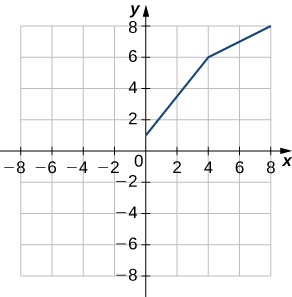40. Use the following graph to evaluate a. $f^{\prime}(-3)$ and b. $f^{\prime}(1.5)$.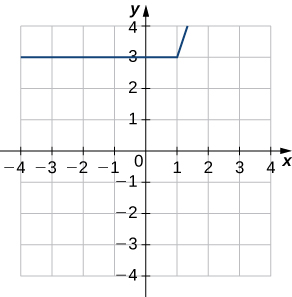For the following exercises, use the limit definition of derivative to show that the derivative does not exist at $x=a$ for each of the given functions.

41. $f(x)=x^{1/3}, \, x=0$

42. $f(x)=x^{2/3}, \, x=0$

43. $f(x)=\begin{cases} 1 & \text{if} \, x<1 \\ x & \text{if} \, x \ge 1 \end{cases}, \, x=1$

44. $f(x)=\frac{|x|}{x}, \, x=0$

45. [T] The position in feet of a race car along a straight track after $t$ seconds is modeled by the function $s(t)=8t^2-\frac{1}{16}t^3$.

1. Find the average velocity of the vehicle over the following time intervals to four decimal places:
1. [4, 4.1]
2. [4, 4.01]
3. [4, 4.001]
4. [4, 4.0001]
2. Use a. to draw a conclusion about the instantaneous velocity of the vehicle at $t=4$ seconds.

46. [T] The distance in feet that a ball rolls down an incline is modeled by the function $s(t)=14t^2$, where $t$ is seconds after the ball begins rolling.

1. Find the average velocity of the ball over the following time intervals:
1. [5, 5.1]
2. [5, 5.01]
3. [5, 5.001]
4. [5, 5.0001]
2. Use the answers from a. to draw a conclusion about the instantaneous velocity of the ball at $t=5$ seconds.

47. Two vehicles start out traveling side by side along a straight road. Their position functions, shown in the following graph, are given by $s=f(t)$ and $s=g(t)$, where $s$ is measured in feet and $t$ is measured in seconds.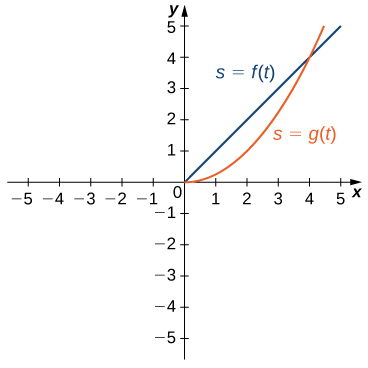1. Which vehicle has traveled farther at $t=2$ seconds?
2. What is the approximate velocity of each vehicle at $t=3$ seconds?
3. Which vehicle is traveling faster at $t=4$ seconds?
4. What is true about the positions of the vehicles at $t=4$ seconds?

48. [T] The total cost $C(x)$, in hundreds of dollars, to produce $x$ jars of mayonnaise is given by $C(x)=0.000003x^3+4x+300$.

1. Calculate the average cost per jar over the following intervals:
1. [100, 100.1]
2. [100, 100.01]
3. [100, 100.001]
4. [100, 100.0001]
2. Use the answers from a. to estimate the average cost to produce 100 jars of mayonnaise.

49. [T] For the function $f(x)=x^3-2x^2-11x+12$, do the following.

1. Use a graphing calculator to graph $f$ in an appropriate viewing window.
2. Use the ZOOM feature on the calculator to approximate the two values of $x=a$ for which $m_{\tan}=f^{\prime}(a)=0$.

50. [T] For the function $f(x)=\frac{x}{1+x^2}$, do the following.

1. Use a graphing calculator to graph $f$ in an appropriate viewing window.
2. Use the ZOOM feature on the calculator to approximate the values of $x=a$ for which $m_{\tan}=f^{\prime}(a)=0$.

51. Suppose that $N(x)$ computes the number of gallons of gas used by a vehicle traveling $x$ miles. Suppose the vehicle gets 30 mpg.

1. Find a mathematical expression for $N(x)$.
2. What is $N(100)$? Explain the physical meaning.
3. What is $N^{\prime}(100)$? Explain the physical meaning.

52. [T] For the function $f(x)=x^4-5x^2+4$, do the following.

1. Use a graphing calculator to graph $f$ in an appropriate viewing window.
2. Use the $\text{nDeriv}$ function, which numerically finds the derivative, on a graphing calculator to estimate $f^{\prime}(-2), \, f^{\prime}(-0.5), \, f^{\prime}(1.7)$, and $f^{\prime}(2.718)$.

53. [T] For the function $f(x)=\frac{x^2}{x^2+1}$, do the following.

1. Use a graphing calculator to graph $f$ in an appropriate viewing window.
2. Use the $\text{nDeriv}$ function on a graphing calculator to find $f^{\prime}(-4), \, f^{\prime}(-2), \, f^{\prime}(2)$, and $f^{\prime}(4)$.

## Glossary

derivative
the slope of the tangent line to a function at a point, calculated by taking the limit of the difference quotient, is the derivative
difference quotient
of a function $f(x)$ at $a$ is given by

$\frac{f(a+h)-f(a)}{h}$ or $\frac{f(x)-f(a)}{x-a}$
differentiation
the process of taking a derivative
instantaneous rate of change
the rate of change of a function at any point along the function $a$, also called $f^{\prime}(a)$, or the derivative of the function at $a$